• 5.78MB weixin_38590309 2021-05-22 12:19:00
• Matlab之最近邻插值'nearest' matlab

最近邻插值’nearest’ 什么是最近邻插值？我的理解是它就是用来对新的像素进行赋值的一种方法。 我为了能方便快速的弄清楚它对图像像素的具体操作，我用的是4×4像素的图像，通过imresize()函数来展示。 ...

最近邻插值 ‘nearest’
什么是最近邻插值？我的理解是它就是用来对新的像素进行赋值的一种方法。
为了能方便快速的弄清楚它对图像像素的具体操作，我用的是4×4像素的图像，通过imresize()函数来展示。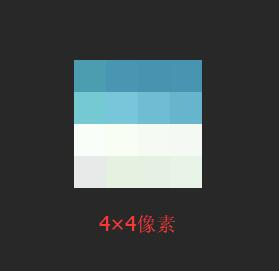对该图像分别缩小0.4倍和0.6倍,因为处理对象太小，图片显示看不到，得到的矩阵如下图所示：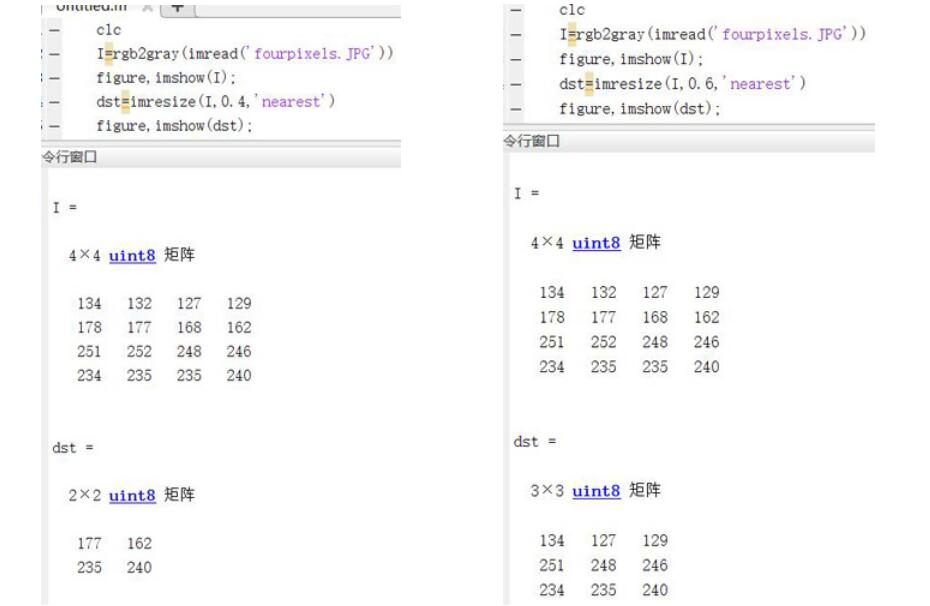这就是用最近邻插值的方法得到2×2和3×3的新矩阵,这里以3×3矩阵来分析。将4×4矩阵用坐标表示：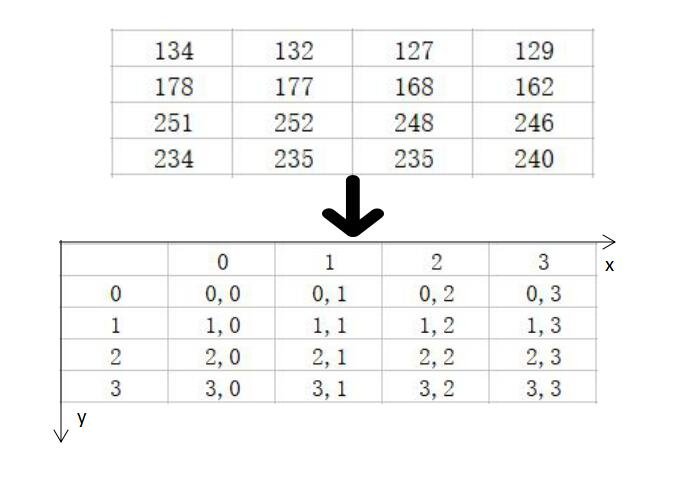压缩0.6倍，变为4×0.6=2.4，要取3，变为3×3大小矩阵，具体变换过程如下图：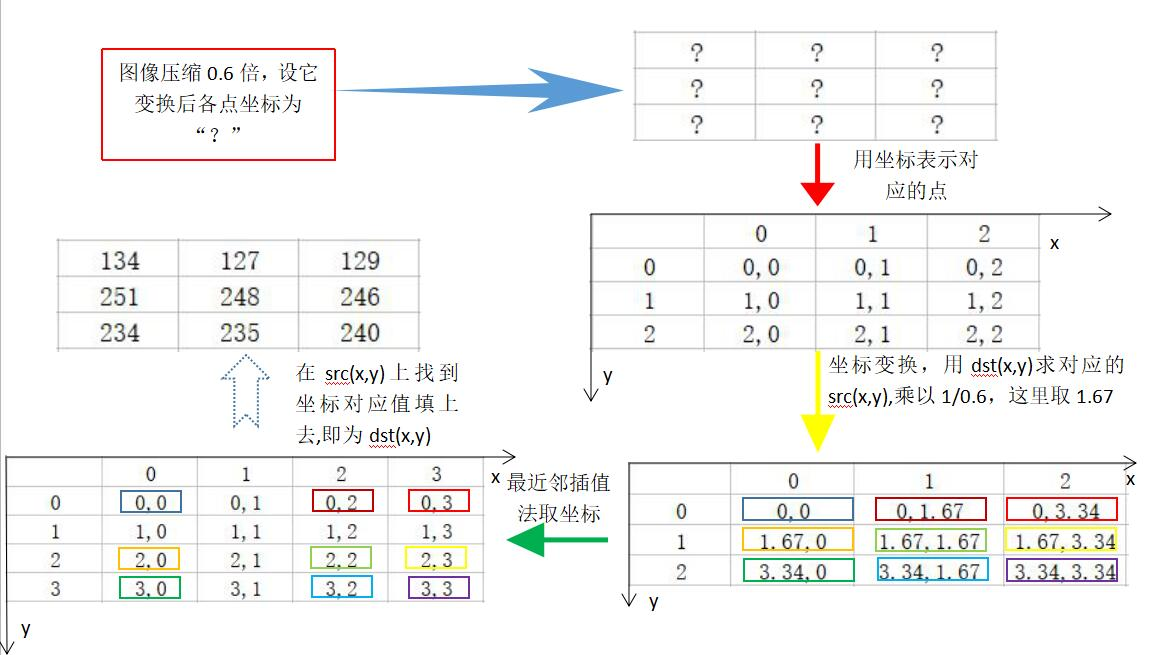这里的坐标对应变换要说明一下，设原图坐标为src(x,y)，变换后的坐标为dst(x,y),放大倍数为S，由dst(x,y)=src(x,y)×S得到src(x,y)=dst(x,y)×1/S。
这种方法就是最近邻插值了，找最近的点的坐标(四舍五入)值赋给目标图像。放大倍数大于1的话其实对像素的操作是一样的原理。

展开全文Bryan_QAQ 2017-12-11 21:52:50
• 2KB wuruihai 2019-04-01 21:47:33
• KD Tree Nearest Neighbor and Range Search：KD Tree 范围和最近邻搜索。-matlab开发 matlab

209KB weixin_38629303 2021-05-31 01:44:05
• Weighted K-Nearest Neighbors (WKNN)：加权 K-Nearest Neighbors (WKNN) 分类器-matlab开发 matlab

1.73MB weixin_38618140 2021-05-29 04:51:24
• 图像插值-最近邻插值（nearest） matlab

最近邻插值（nearest） ...本程序是matlab写的一个my_nearest函数，与matlab自有的nearest函数相对应。可以实现任意倍数的放大（其中涉及边界索引的问题）。 matlab代码： function [dst]=my_nearest(sr

最近邻插值（nearest）
本文将未做插值的原始图像称作源图像，源图像插值缩放K倍后的图像称作目标图像。
以下标识符的意义：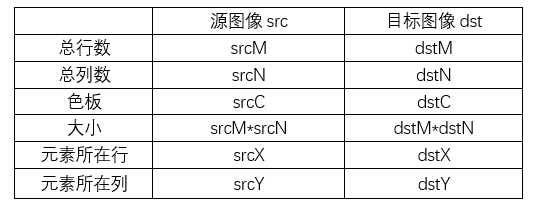1. 算法
该算法不凭空设定元素点的值，而是选择源图像中最近邻像素点来填充目标图像。
那么，目标元素点所选择的源图像元素点位置应为：
srcX=round(dstX/K)
srcY=round(dstY/K)
其中，round表示将值四舍五入为整数
2. 实现
本程序是matlab写的一个my_nearest函数，与matlab自有的imresize的nearest型函数相对应。可以实现任意倍数的放大（其中涉及边界索引的问题需要注意，这在双线性和双三次插值中也会遇到）。

matlab代码：

function [dst]=my_nearest(src,K)
%最近邻法插值
%输入：源图像src，放大倍数K
%输出：目标图像矩阵dst

[srcM,srcN,srcC]=size(src);%读取源图像元素点的行列数及色板数
dstM=round(K*srcM);%该处仍要确保当放大倍数K非整数时目标图像大小为整数
dstN=round(K*srcN);

%使用class将数据类型统一，目标图像初始化
dst=ones(dstM,dstN,srcC,class(src));

%逐像素点赋值
for dstX=1:dstM
for dstY=1:dstN
for dstC=1:srcC
srcX=round(dstX/K);
srcY=round(dstY/K);
srcX(srcX>srcN)=srcN; %防止索引源图像界外位置,该函数很耗时但简洁
srcX(srcX<1)=1;
srcY(srcY>srcM)=srcM ;
srcY(srcY<1)=1;
dst(dstX,dstY,dstC)=src(srcX,srcY,dstC);
end
end
end
end

1. 评估
分别使用本文函数my_nearest函数和matlab的imresize函数中的neaerest型对lena图进行2倍放大，并计时。

test代码：

close all
figure
imshow(A);
figure
tic
imshow(my_nearest(A,2));
toc
figure
tic
imshow(imresize(A,2,'nearest'));
toc

结果：细节：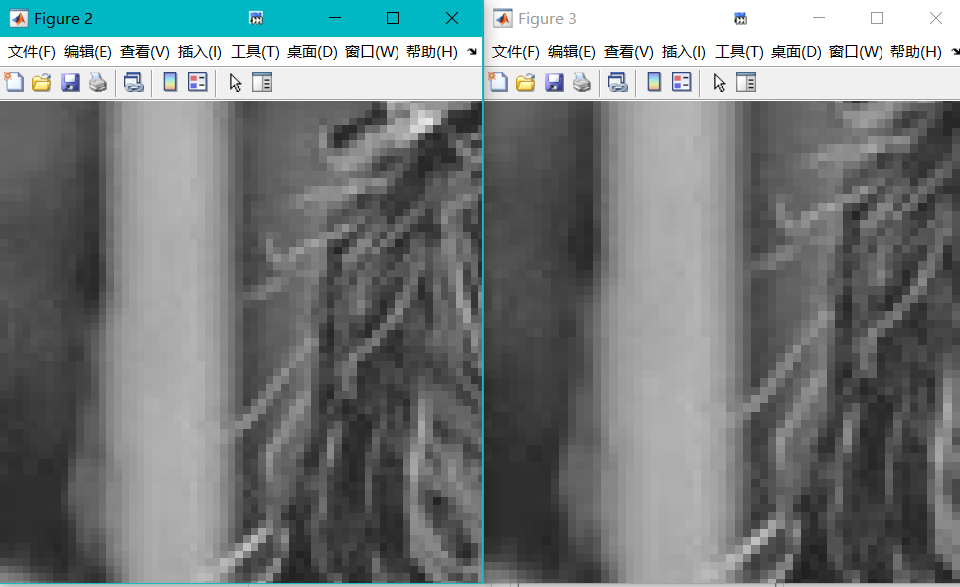耗时对比：综上，两者效果无差别，但my_nearest函数更慢，这是因为matlab自身使用矩阵运算，速度更快。
该算法速度快，但效果不如双线性插值和双三次插值好。
附：
双线性插值
双三次插值

展开全文kill2013110 2020-08-20 15:23:40
• matlab中nearest,ind2sub... python matlab

python 实现matlabnearest,ind2sub

matlab命令

nearest

nearest 返回 半径范围内最近的邻点 –参考链接

语法说明
nodeIDs = nearest(G,s,d)nodeIDs = nearest(G,s,d) 返回图形 G 中与节点 s 的距离在 d 之内的所有节点。如果图进行了加权（即 G.Edges 包含变量 Weight），则这些权重用作沿图中各边的距离。否则，所有边距离都视为 1。
nodeIDs = nearest(G,s,d,Name,Value)nodeIDs = nearest(G,s,d,Name,Value) 使用一个或多个名称-值对组参数指定的其他选项。例如，如果 G 是加权图，则 nearest(G,s,d,‘Method’,‘unweighted’) 将忽略图 G 中的边权重，而将所有边权重视为 1。
[nodeIDs, dist] = nearest(___)[nodeIDs,dist] = nearest(___) 还返回与每个最近邻点之间的距离，因此 dist(j) 是从源节点 s 到节点 nodeIDs(j) 的距离。您可以使用上述语法中的任何输入参数组合。

最近节点示例

s = [1 1 1 1 1 2 2 2 3 3 3 3 3];
t = [2 4 5 6 7 3 8 9 10 11 12 13 14];
weights = randi([1 10],1,13);
G = graph(s,t,weights);
p = plot(G,'Layout','force','EdgeLabel',G.Edges.Weight);确定哪些节点在节点 1 周围半径为 15 的范围内。

nn = nearest(G,1,15)

% Output
nn = 9×1

5
7
2
3
4
6
8
12
9

源节点以绿色突出显示，最近的邻点以红色突出显示。（权值采用随机数，故每次输出的图像会不一样）

highlight(p,1,'NodeColor','g')
highlight(p,nn,'NodeColor','r')最近节点的距离示例

ss = [1 1 1 2 2 6 6 7 7 3 3 9 9 4 4 11 11 8];
t = [2 3 4 5 6 7 8 5 8 9 10 5 10 11 12 10 12 12];
weights = [10 10 10 10 10 1 1 1 1 1 1 1 1 1 1 1 1 1];
G = graph(s,t,weights);
p = plot(G,'EdgeLabel',G.Edges.Weight)确定哪些节点在节点 3 周围半径为 5 的范围内，并返回到每个节点的距离。

[nn,dist] = nearest(G,3,5)
highlight(p,3,'NodeColor','g')
highlight(p,nn,'NodeColor','r')

% Output
nn = 9×1

9
10
5
11
4
7
12
6
8

dist = 9×1

1
1
2
2
3
3
3
4
4ind2sub

ind2sub 将线性索引转换为下标 - 参考链接

语法说明
[row,col] = ind2sub(sz,ind)返回数组 row 和 col，其中包含与大小为 sz 的矩阵的线性索引 ind 对应的等效行和列下标。此处，sz 是包含两个元素的向量，其中 sz(1) 指定行数，sz(2) 指定列数。
[I1,I2,...,In] = ind2sub(sz,ind)返回 n 个数组 I1,I2,…,In，其中包含与大小为 sz 的多维数组的线性索引 ind 对应的等效多维下标。此处，sz 是包含 n 个元素的向量，用于指定每个数组维度的大小。

将矩阵的线性索引转换为下标

将 3×3 矩阵的线性索引 [3 4 5 6] 转换为行和列下标。从线性索引到下标（按位置进行索引）的映射如下所示。创建输入向量并执行转换。

ind = [3 4 5 6];
sz = [3 3];
[row,col] = ind2sub(sz,ind)

row = 1×4

3     1     2     3

col = 1×4

1     2     2     2

将线性索引转换为三维数组的下标

将 2×2×2 数组的线性索引 [3 4 5 6] 转换为下标。2×2×2 数组中从线性索引到下标（按位置进行索引）的映射如下所示。创建输入向量并执行转换。

ind = [3 4 5 6];
sz = [2 2 2];
[I1,I2,I3] = ind2sub(sz,ind)

I1 = 1×4

1     2     1     2

I2 = 1×4

2     2     1     1

I3 = 1×4

1     1     2     2

python 实现matlab中的ind2sub

import math
def ind2sub(array_shape, ind):
'''
:param array_shape: 可以为一维，二维，三维，如, [3,3], [2,2,2]
:param ind:  索引一维数组，如 ind=[3, 4, 5, 6, 7, 9, 10, 11]
:return:  转换后的下标值
'''
if len(array_shape) == 1:
rows = ind
cols = [1 for i in range(len(ind))]
return rows, cols
elif len(array_shape) == 2:
rows, cols = [], []
for i in ind:
rows.append(array_shape if i % array_shape == 0 else i % array_shape)  # 取余
cols.append(math.ceil(i / array_shape))
return rows, cols
elif len(array_shape) == 3:
rows, cols, pages = [], [], []
for i in ind:
pageSize = array_shape * array_shape
page = math.ceil(i / pageSize)   # 确定在第几页
remainder = pageSize if i % pageSize == 0 else i % pageSize   # 余数
rows.append(array_shape if remainder % array_shape == 0 else remainder % array_shape)
cols.append(math.ceil(remainder / array_shape))
pages.append(page)
return rows, cols, pages
else:
print('暂时无法将索引转换为下标！')

参考链接

展开全文weixin_31948131 2020-12-29 14:22:27
• 172KB weixin_38689551 2021-05-21 18:59:04
• 采用matlab内部的函数来实现最邻近插值、双线性插值和双三次插值，实现2倍放大。 代码如下： I=imread('lena.bmp'); imshow(I); I1=imresize(I,2,'nearest'); figure imshow(I1); I2=imresize(I,2,'...

采用matlab内部的函数来实现最邻近插值、双线性插值和双三次插值，实现2倍放大。

代码如下：

imshow(I);
I1=imresize(I,2,'nearest');
figure
imshow(I1);
I2=imresize(I,2,'bilinear');
figure
imshow(I2);
I3=imresize(I,2,'bicubic');
figure
imshow(I3);

转载于:https://www.cnblogs.com/fsp2008/p/10640505.html

展开全文weixin_30537451 2019-04-02 09:16:00
• 663B weixin_38744435 2019-08-23 19:34:32
• [数字图像处理]最近邻插值和双线性插值（nearest neighbor interpolation and bilinear interpolation）... 数字图像处理 matlab

zzx2015 2019-03-12 23:36:05
• 2.61MB weixin_42669344 2021-09-29 16:15:04
• 通过Matlab对龙格函数作nearest、linear、spline这三种多项式插值 matlab

weixin_44359479 2021-04-11 00:34:11
• shixin_0125 2020-01-16 19:54:13
• 756KB weixin_42696271 2021-09-11 11:35:42
• Nearest Neighbor Descent (NN-Descent)：精确计算k-最近邻或有效地近似k-最近邻。-matlab开发 matlab

57KB weixin_38521169 2021-05-31 20:03:25
• k-Nearest Neighbors (kNN) Regressor：使用 kNN 进行简单和多重回归-matlab开发 matlab

55KB weixin_38652058 2021-05-31 21:30:25
• 用于图像分割的 K-Nearest Neighbors：K-Nearest Neighbors 算法的实现。-matlab开发 matlab

3KB weixin_38631960 2021-05-30 11:09:34
• 168KB shiyimin1 2018-04-29 16:50:39
• 1KB pudn01 2021-08-11 18:39:49
• weixin_31094177 2021-04-22 10:01:13
• 通过 k-Nearest Neighbour 进行分类的教程：使用 k-Nearest Neighbor 对 3 类问题进行一维矩阵分类的基础... matlab

4KB weixin_38694699 2021-05-29 07:23:36
• Nearest positive semideterminance covariance matrix：找到与非正半定对称矩阵最近的正半定矩阵-matlab... matlab

2KB weixin_38735887 2021-06-01 12:22:31
• Find Nearest Neighbors on Sphere：与 GridSphere 函数一起使用以在测地线网格上查找最近的查询点。... matlab

25KB weixin_38643127 2021-05-30 07:39:15
• 4KB john_xia 2020-07-24 15:59:55
• weixin_36271649 2021-04-18 09:15:04
• 15.94MB weixin_38747025 2021-05-28 08:48:46
• weixin_36308612 2021-04-20 00:47:33
• facetosea1 2018-10-10 20:38:59...

matlabnearestmatlab 订阅